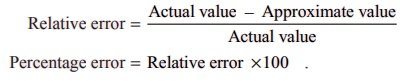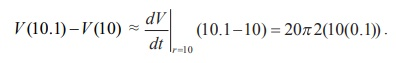Home | | Maths 12th Std | Errors: Absolute Error, Relative Error, and Percentage Error

# Errors: Absolute Error, Relative Error, and Percentage Error

When we are approximating a value, there occurs an error. In this section, we consider the error, which occurs by linear approximation, given by (4).

Errors: Absolute Error, Relative Error, and Percentage Error

When we are approximating a value, there occurs an error. In this section, we consider the error, which occurs by linear approximation, given by (4). We shall consider different types of errors. Taking h = x ŌłÆ x0 , we get x = x0 + h , then (5) becomes

E ( h) = f (x0 + h ) ŌłÆ f (x0 ) ŌłÆ f ŌĆ▓(x0 )h .          ... (6)

Note that E(0) = 0 and as we have already observed limhŌåÆ0 E ( h) = 0 follows from the continuity of f at x0 . In addition, if f is differentiable, then from (1), it follows thatThus when f is differentiable at x0 , then the above equation shows that E ( h) actually approaches zero faster than h approaching zero. Now, we define

### Definition 8.2

Suppose that certain quantity is to be determined. ItŌĆÖs exact value is called the actual value. Some times we obtain its approximate value through some approximation process. In this case, we define

Absolute error = Actual value ŌłÆ Approximate value.

So (6) gives the absolute error that occurs by a linear approximation. Let us look at an example illustrating the use of linear approximation.

### Example 8.2

Use linear approximation to find an approximate value of ŌłÜ9.2 without using a calculator.

### Solution

We need to find an approximate value of ŌłÜ9.2 using linear approximation. Now by (3), we have f (x0 + ╬öx ) Ōēł f (x0 ) + f ŌĆ▓(x0 ) ╬öx . To do this, we have to identify an appropriate function f , a point x0 and ╬öx . Our choice should be such that the right side of the above approximate equality, should be computable without the help of a calculator. So, we choose f (x) = ŌłÜx , x0 = 9 and ╬öx = 0.2 . Then, f ŌĆ▓(x0) = 1/2ŌłÜ9 and hence

ŌłÜ9.2 Ōēł f (9) + f ŌĆ▓(9)(0.2) = 3 + 0.2/6 = 3.03333 .

Now if we use a calculator, just to compare, we find ŌłÜ9.2 = 3.03315 . We see that our approximation is accurate to three decimal places and the error is 3.03315 ŌłÆ 3.03333 = ŌłÆ0.00018 . [Also note that one could choose f (x) = ŌłÜ(1+ x) , x0 = 8 and ╬öx = 0.2 . So the choice of f and x0 are not necessarily unique].

So in the above example, the absolute error is 3. 03315 3.03333 0.00018 . Note that the absolute error says how much the error; but it does not say how good the approximation is. For instance, let us consider two simple cases.

Case 1 : Suppose that the actual value of something is 5 and its approximated value is 4 , then the absolute error is 5 ŌłÆ 4 = 1 .

Case 2 : Suppose that the actual value of something is 100 and its approximated value is 95. In this case, the absolute error is 100 ŌłÆ 95 = 5 . So the absolute error in the first case is smaller when compared to the second case.

Among these two approximations, which is a better approximation; and why? The absolute error does not give a clear picture about whether an approximation is a good one or not. On the other hand, if we calculate relative error or percentage of error (defined below), it will be easy to see how good an approximation is. If the actual value is zero, then we do know how close our approximate answer is to the actual value. So if the actual value is not zero, then we define,

Definition 8.3

If the actual value is not zero, then

Relative error = [ Actual value ŌłÆ Approximate value ] / Actual value

Percentage error = Relative error ├Ś100 .Note: Absolute error has unit of measurement where as relative error and percentage error are units free.

Note that, in the case of the above examples,

In the first case

The relative error = 1/5 = 0.2 ; and the percentage error = 1/5 ├Ś100 = 20% .

In the second case

The relative error = 5/100 ; and the percentage error = 5/100 ├Ś100 = 5%.

So the second approximation is a better approximation than the first one. Note that, in order to calculate the relative error or the percentage error one should know the actual value of what we are approximating.

Let us consider some examples.

Example 8.3

Let us assume that the shape of a soap bubble is a sphere. Use linear approximation to approximate the increase in the surface area of a soap bubble as its radius increases from 5 cm to 5.2 cm. Also, calculate the percentage error.

Solution

Recall that surface area of a sphere with radius r is given by S ( r) = 4ŽĆ r2 . Note that even though we can calculate the exact change using this formula, we shall try to approximate the change using the linear approximation. So, using (4), we have

Change in the surface area = S (5.2) ŌłÆ S(5) Ōēł SŌĆ▓(5)(0.2)

= 8ŽĆ(5)( 0 .2) = 8ŽĆ cm2

Exact calculation of the change in the surface gives

S (5.2) ŌłÆ S(5) = 108.16ŽĆ - 100 ŽĆ = 8.16cm2 .

Percentage error = relative error ├Ś 100 = { 8.16ŽĆŌłÆ8ŽĆ / 8.16ŽĆ  } ├Ś 100 = 1.9607%

Example 8.4

A right circular cylinder has radius r = 10 cm. and height h = 20 cm. Suppose that the radius of the cylinder is increased from 10 cm to 10.1 cm and the height does not change. Estimate the change in the volume of the cylinder. Also, calculate the relative error and percentage error.

Solution

Recall that volume of a right circular cylinder is given by V = ŽĆ r2h , where r is the radius and h is the height. So we have V ( r) = ŽĆr2h = 20ŽĆr2.Thus the estimate for the change in the volume is 40ŽĆ cm3.

Exact calculation of the volume change gives

V (10.1) ŌłÆV (10) = 2040.2ŽĆ ŌłÆ 2000ŽĆ = 40.2ŽĆ cm3.

So relative error = (40.2ŽĆŌłÆ40ŽĆ) / (40.2ŽĆ)  = 1 / 201 = 0.00497 ; and hence

the percentage error = relative error ├Ś 100 = [1/201] ├Ś 100 = 0.497% .

Tags : Linear Approximation | Mathematics , 12th Maths : UNIT 8 : Differentials and Partial Derivatives
Study Material, Lecturing Notes, Assignment, Reference, Wiki description explanation, brief detail
12th Maths : UNIT 8 : Differentials and Partial Derivatives : Errors: Absolute Error, Relative Error, and Percentage Error | Linear Approximation | Mathematics